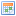Lua: InterpolationDescription: How to use interpolationOriginal Author: Brian NevecCreated: June 19, 2010

## Introduction

In this article I'm going to discuss how to use several interpolation techniques in GMod. This article will only cover the basics, but will explain everything you need to know to use interpolation in your code.

Interpolation is essentially a way of approximating data using known control points. This will explain what interpolation is more thoroughly.

In games interpolation is mostly used to create smooth transitions between values and create 2D and 3D curves.

### Method 1

A commonly used method is to simply increment a value until it reaches its target.

```-- execute this code only once

-- the starting value
local startVal = 5;
-- the target value
local endVal = 10;
-- the speed; increment by this every second
local speed = 2;

-- the incrementing value
local value = 0;

-- execute this code every frame

-- increment the value by speed
-- in order for the value to change independently of FPS,
-- it is required to multiply the speed constant by the time it took to draw the last frame
value = value + speed * FrameTime( );

-- make sure the value only goes from startVal to endVal
value = math.Clamp( value, startVal, endVal );```

This method is a form of linear interpolation; the value changes at a constant rate. Decrementing is as easy as either changing from '+' to '-' or changing the speed to a negative value. The result will be the same.

### Method 2

The previous method allowed you to specify the speed. This method allows you to specify the time it will take for the value to reach its target. This method may be a bit harder to implement.

```-- execute this only once

-- start
local startTime = 0;
-- the time it will take
-- starting value
local startVal = 5;
-- target value
local endVal = 10;

-- the value
local value = startVal;

-- execute this when you want the value to start changing

-- at this time value will be startVal
startTime = CurTime( );

-- execute this every frame

-- this value represents change
-- when it is zero, value will be startVal
-- when it is one, value will be endVal
local fraction = ( CurTime( ) - startTime ) / lifeTime;
fraction = math.Clamp( fraction, 0, 1 );

-- GMod provides us with a function that performs linear interpolation
-- we calculate the fraction above
-- we do not need to do any clamping
value = Lerp( fraction, startVal, endVal );```

### Method 3

This is pretty much the most simplest method. However, the resulting change slows down when close to the target, so this method is not a form of linear interpolation.

```-- execute this only once

-- the value
local value = 0;
-- the target value
local targetValue = 10;
-- the speed
-- this may not represent change per second
local speed = 20;

-- execute this every frame

-- linearly interpolate from whatever value is right now to targetValue
value = Lerp( speed * FrameTime( ), value, targetValue );```

## Summary

These three methods each have their own pros and cons. It's up to you to choose the right one.

## Basics of curves

More complex interpolation methods give you more control over the resulting value. You can specify more properties as well as control points. This article only explains the Bézier curve, but most other curves work in a similar way.

The Bézier curve is described in detail here.

In the examples provided below, the variable 'a' is a fraction between 0 and 1 and the variable 'b' is equal to '1 - a'.

Expanding the expression '(a + b)^n' will give you essentially these 'control values' by which to multiply your control points. 'n' represents the number of control points - 1.

### Linear curve

A linear Bézier curve is a straight line. The expression we need to expand is '(a + b)^1', which is equal to 'a + b'.

`value = cp1 * a + cp2 * b;`

A quadratic Bézier curve is a curve represented by three control points. The expression is as follows: `(a + b)^2 = a^2 + 2ab + b^2`

As you can see, three monomials (or control values) are the result of the expansion.

`value = cp1 * a^2 + cp2 * 2ab + cp3 * b^2;`

### Other Bézier curves

Other Bézier curves can be achieved by simply increasing the power. This approach, however, provides you with only a finite number of control points. The more universal method is described in the wiki article under the title 'Constructing Bézier curves'.